# Swimlane

4 Apr 202224 minutes to read

Swimlane is a type of diagram nodes, which is typically used to visualize the relationship between a business process and the department responsible for it by focusing on the logical relationships between activities.

## Create a swimlane

To create a swimlane, the type of shape should be set as `swimlane`. By Default swimlane’s are arranged vertically.

Header was the primary element for swimlanes. The `header` property of swimlane allows to define its textual description and to customize its appearance.

By using this header, the swimlane interaction will be performed, like selection, dragging, etc.

``````<ejs-diagram id="container" width="100%" height="700px">
<e-diagram-nodes>
<e-diagram-node id='node1' offsetX="100" offsetY="100" width="100" height="100" borderWidth="2">
<e-node-style fill="darkcyan", strokeColor="black" strokeWidth="2"></e-node-style>
</e-diagram-node>
</e-diagram-nodes>
</ejs-diagram>``````
``````using System;
using System.Collections.Generic;
using System.Linq;
using System.Web;
using System.Web.Mvc;
using Syncfusion.EJ2.Diagrams;
using System.Drawing;

namespace EJ2MVCSampleBrowser.Controllers.Diagram {
public partial class DiagramController: Controller {
// GET: Nodes
public ActionResult Nodes() {
List < DiagramNode > nodes = new List < DiagramNode > ();
List < DiagramNodeAnnotation > Node1 = new List < DiagramNodeAnnotation > ();
Content = "node1", Style = new DiagramTextStyle() {
Color = "White", StrokeColor = "None"
}
});
List<Lane> Lanes = new List<Lane>();
List<Phase> Phases = new List<Phase>();
swimlane.Shape  =  new SwimLane(){
Type = "SwimLane",
PhaseSize = 20,
{
Annotation = new DiagramNodeAnnotation() { Content = "ONLINE PURCHASE STATUS" },
Height = 50,
Orientation = "Horizontal",
Style = new DiagramTextStyle() { FontSize = 11 }
},
Lanes = Lanes,
Phases = Phases
};
ViewBag.nodes = nodes;
return View();
}
}
public class Node: DiagramNode {
public string text;
}
}``````

The height and width of swimlane header can be customized with `weight` and `height` properties of swimlane header. Set fill color of header by using the `style` property. The orientation of swimlane can be customized with the `orientation` property of the header.

By default the swimlane orientation has Horizontal.

``````<ejs-diagram id="container" width="100%" height="700px">
<e-diagram-nodes>
<e-diagram-node id='node1' offsetX="100" offsetY="100" width="100" height="100" constraints="Shadow">
</e-diagram-node>
</e-diagram-nodes>
</ejs-diagram>``````
``````using System;
using System.Collections.Generic;
using System.Linq;
using System.Web;
using System.Web.Mvc;
using Syncfusion.EJ2.Diagrams;
using System.Drawing;

namespace EJ2MVCSampleBrowser.Controllers.Diagram {
public partial class DiagramController: Controller {
// GET: Nodes
public ActionResult Nodes() {
List < DiagramNode > nodes = new List < DiagramNode > ();
List < DiagramNodeAnnotation > Node1 = new List < DiagramNodeAnnotation > ();
Content = "node1", Style = new DiagramTextStyle() {
Color = "White", StrokeColor = "None"
}
});
List<Lane> Lanes = new List<Lane>();
List<Phase> Phases = new List<Phase>();
swimlane.Shape  =  new SwimLane(){
Type = "SwimLane",
PhaseSize = 20,
{
Annotation = new DiagramNodeAnnotation() { Content = "ONLINE PURCHASE STATUS" },
Height = 50,
Orientation = "Horizontal",
Style = new DiagramTextStyle() { FontSize = 11 ,fill ="red"}
},
Lanes = Lanes,
Phases = Phases
};
ViewBag.nodes = nodes;
return View();
}
}
public class Node: DiagramNode {
public string text;
}
}``````

### Dynamic customization of swimlane header

You can customize the swimlane header style and text properties dynamically.

``````let lane : nodeModel = diagram.nodes;
diagram.dataBind();``````

Diagram provides the support to edit swimlane headers at runtime. The header editing is achieved by double click event. Double clicking the header label will enable the editing.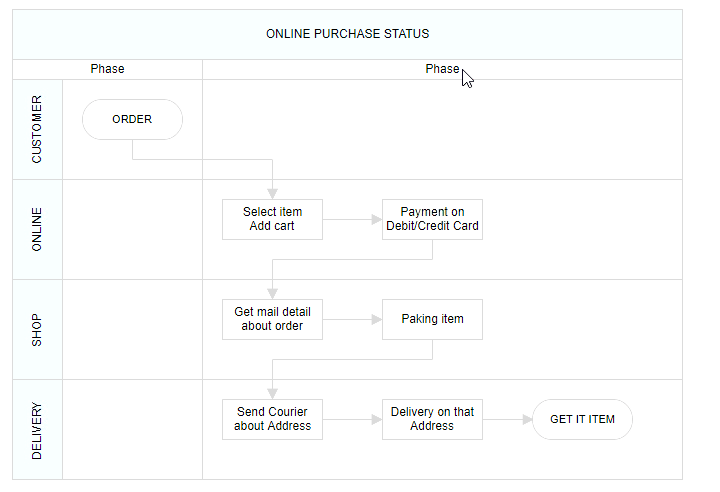## Lanes

Lane is a functional unit or a responsible department of a business process that helps to map a process within the functional unit or in between other functional units.

The number of `lanes` can be added to swimlane. The lanes are automatically stacked inside swimlane based on the order they are added.

### Create an empty lane

• The lanes `id` is used to define the name of the lane and its further used to find the lane at runtime and do any customization.

• The additional information to the lane is provided by using the `addInfo` property of the lane.

``````<ejs-diagram id="container" width="100%" height="700px">
<e-diagram-nodes>
<e-diagram-node id='node1' offsetX="100" offsetY="100" width="100" height="100" constraints="Shadow">
</e-diagram-node>
</e-diagram-nodes>
</ejs-diagram>``````
``````using System;
using System.Collections.Generic;
using System.Linq;
using System.Web;
using System.Web.Mvc;
using Syncfusion.EJ2.Diagrams;
using System.Drawing;

namespace EJ2MVCSampleBrowser.Controllers.Diagram {
public partial class DiagramController: Controller {
// GET: Nodes
public ActionResult Nodes() {
List < DiagramNode > nodes = new List < DiagramNode > ();
List < DiagramNodeAnnotation > Node1 = new List < DiagramNodeAnnotation > ();
Content = "node1", Style = new DiagramTextStyle() {
Color = "White", StrokeColor = "None"
}
});
List<Lane> Lanes = new List<Lane>();
List<Phase> Phases = new List<Phase>();
swimlane.Shape  =  new SwimLane(){
Type = "SwimLane",
PhaseSize = 20,
{
Annotation = new DiagramNodeAnnotation() { Content = "ONLINE PURCHASE STATUS" },
Height = 50,
Orientation = "Horizontal",
Style = new DiagramTextStyle() { FontSize = 11 ,fill ="red"}
},
Lanes = Lanes,
Phases = Phases
};
ViewBag.nodes = nodes;
return View();
}
}
public class Node: DiagramNode {
public string text;
}
}``````

• The `header` property of lane allows to textually describe the lane and to customize the appearance of the description.
``````<ejs-diagram id="container" width="100%" height="700px">
<e-diagram-nodes>
<e-diagram-node id='node1' offsetX="100" offsetY="100" width="100" height="100" borderWidth="2">
<e-node-style fill="darkcyan", strokeColor="black" strokeWidth="2"></e-node-style>
</e-diagram-node>
</e-diagram-nodes>
</ejs-diagram>``````
``````using System;
using System.Collections.Generic;
using System.Linq;
using System.Web;
using System.Web.Mvc;
using Syncfusion.EJ2.Diagrams;
using System.Drawing;

namespace EJ2MVCSampleBrowser.Controllers.Diagram {
public partial class DiagramController: Controller {
// GET: Nodes
public ActionResult Nodes() {
List < DiagramNode > nodes = new List < DiagramNode > ();
List < DiagramNodeAnnotation > Node1 = new List < DiagramNodeAnnotation > ();
Content = "node1", Style = new DiagramTextStyle() {
Color = "White", StrokeColor = "None"
}
});
List<Lane> Lanes = new List<Lane>();
{
Id = "stackCanvas1",
Height = 100,
{
Annotation = new DiagramNodeAnnotation() { Content = "Consumer" },
Width = 50,
},
});
List<Phase> Phases = new List<Phase>();
swimlane.Shape  =  new SwimLane(){
Type = "SwimLane",
PhaseSize = 20,
{
Annotation = new DiagramNodeAnnotation() { Content = "ONLINE PURCHASE STATUS" },
Height = 50,
Orientation = "Horizontal",
Style = new DiagramTextStyle() { FontSize = 11 ,fill ="red"}
},
Lanes = Lanes,
Phases = Phases
};
ViewBag.nodes = nodes;
return View();
}
}
public class Node: DiagramNode {
public string text;
}
}``````

``````<ejs-diagram id="container" width="100%" height="700px">
<e-diagram-nodes>
<e-diagram-node id='node1' offsetX="100" offsetY="100" width="100" height="100" borderWidth="2">
<e-node-style fill="darkcyan", strokeColor="black" strokeWidth="2"></e-node-style>
</e-diagram-node>
</e-diagram-nodes>
</ejs-diagram>``````
``````using System;
using System.Collections.Generic;
using System.Linq;
using System.Web;
using System.Web.Mvc;
using Syncfusion.EJ2.Diagrams;
using System.Drawing;

namespace EJ2MVCSampleBrowser.Controllers.Diagram {
public partial class DiagramController: Controller {
// GET: Nodes
public ActionResult Nodes() {
List < DiagramNode > nodes = new List < DiagramNode > ();
List < DiagramNodeAnnotation > Node1 = new List < DiagramNodeAnnotation > ();
Content = "node1", Style = new DiagramTextStyle() {
Color = "White", StrokeColor = "None"
}
});
List<Lane> Lanes = new List<Lane>();
{
Id = "stackCanvas1",
Height = 100,
{
Annotation = new DiagramNodeAnnotation() { Content = "Consumer" },
Width = 50,Style = new DiagramTextStyle() { FontSize = 11 ,fill ="red"}
},
});
List<Phase> Phases = new List<Phase>();
swimlane.Shape  =  new SwimLane(){
Type = "SwimLane",
PhaseSize = 20,
{
Annotation = new DiagramNodeAnnotation() { Content = "ONLINE PURCHASE STATUS" },
Height = 50,
Orientation = "Horizontal",
Style = new DiagramTextStyle() { FontSize = 11 ,fill ="red"}
},
Lanes = Lanes,
Phases = Phases
};
ViewBag.nodes = nodes;
return View();
}
}
public class Node: DiagramNode {
public string text;
}
}``````

### Dynamic customization of lane header

You can customize the lane header style and text properties dynamically.

``````let lane : nodeModel = diagram.nodes;
diagram.dataBind();``````

You can add the lane at runtime by using the client side API method called `addLanes`.

To add nodes to lane, you should add `children` collection of the lane.

``````<ejs-diagram id="container" width="100%" height="700px" nodes="@ViewBag.nodes">
</ejs-diagram>``````
``````using System;
using System.Collections.Generic;
using System.Linq;
using System.Web;
using System.Web.Mvc;
using Syncfusion.EJ2.Diagrams;
using System.Drawing;

namespace EJ2MVCSampleBrowser.Controllers.Diagram {
public partial class DiagramController: Controller {
// GET: Nodes
public ActionResult Nodes() {
List<DiagramNode> Nodes = new List<DiagramNode>();
//Create lanes
List<Lane> Lanes = new List<Lane>();
{
Id = "stackCanvas1",
Height = 100,
{
Annotation = new DiagramNodeAnnotation() { Content = "Consumer" },
Width = 50,
},
Children = firstLaneChildren
});

{
Id = "stackCanvas2",
Height = 100,
{
Annotation = new DiagramNodeAnnotation() { Content = "Marketing" },
Width = 50,
},
Children = secondLaneChildren
});

{
Id = "stackCanvas3",
Height = 100,
{
Annotation = new DiagramNodeAnnotation() { Content = "Sales" },
Width = 50,
},
Children = thirdLaneChildren
});

{
Id = "stackCanvas4",
Height = 100,
{
Annotation = new DiagramNodeAnnotation() { Content = "Success" },
Width = 50,
},
Children = fourthLaneChildren
});

//Create phases
List<Phase> Phases = new List<Phase>();
{
Id = "phase1",
Offset = 170,
{
Annotation = new DiagramNodeAnnotation() { Content = "Phase" },
},
});

swimlane.Shape = new SwimLane()
{
Type = "SwimLane",
PhaseSize = 20,
{
Annotation = new DiagramNodeAnnotation() { Content = "SALES PROCESS FLOW CHART" },
Height = 50,
Orientation = "Horizontal",
Style = new DiagramTextStyle() { FontSize = 11 }
},
Lanes = Lanes,
Phases = Phases
};
return View();
}
}
public class Node: DiagramNode {
public string text;
}
}``````

### Dynamically add children to lane

The diagram provides support to add children dynamically.

`````` let node ={
id: 'Order',
shape: { type: 'Path', data: pathData },
annotations: [
{
content: 'ORDER',
style: { fontSize: 11 }
} ],
margin: { left: 60, top: 20 },
height: 40, width: 100
}

let lane : nodeModel = diagram.nodes;
swimlane.shape.lanes.childern=node;
diagram.dataBind();``````

### Resizing lane

• Lane can be resized in the bottom and left direction.
• Lane can be resized by using resize selector of the lane.
• Once you can resize the lane, the swimlane will be resized automatically.
• The lane can be resized by either resizing the selector or the tight bounds of the child object. If the child node moves to the edge of the lane, it can be automatically resized.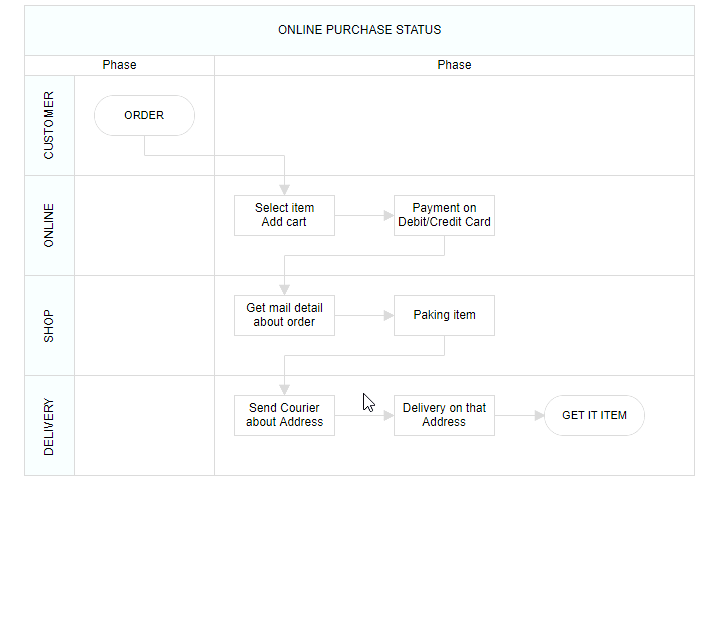### Lane swapping

• Lanes can be swapped using drag the lanes over another lane.
• Helper should intimate the insertion point while lane swapping.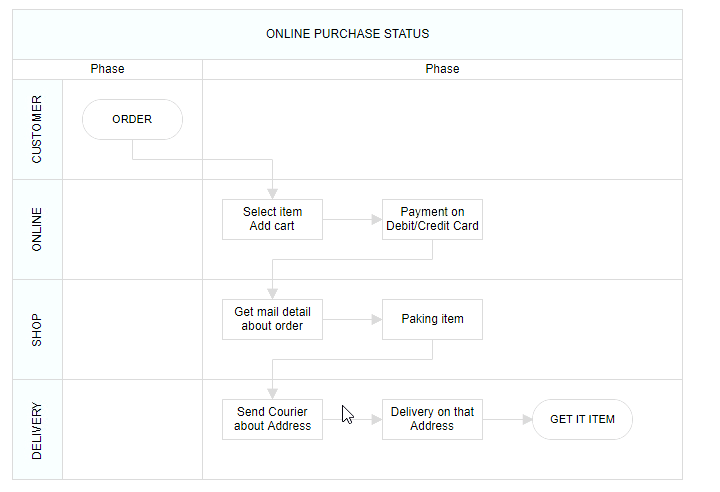### Disable Swimlane Lane swapping

You can disable swimlane lane swapping by using the property called `canMove`.

`````` let node ={
id: 'Order',
shape: { type: 'Path', data: pathData },
annotations: [
{
content: 'ORDER',
style: { fontSize: 11 }
} ],
margin: { left: 60, top: 20 },
height: 40, width: 100,canMove: false
}

let lane : nodeModel = diagram.nodes;
swimlane.shape.lanes.childern=node;
diagram.dataBind();``````

### Resize helper

• The special resize helper will be used to resize the lanes.
• The resize cursor will be available on the left and bottom direction alone.
• Once the lane is resized, the swimlane will be resized automatically.

### Children interaction in lanes

• You can resize the child node within swimlanes.
• You can drag the child nodes within lane.
• Interchange the child nodes from one lane to another lane.
• Drag and drop the child nodes from lane to diagram.
• Drag and drop the child nodes from diagram to lane.
• Based on the child node interactions, the lane size should be updated.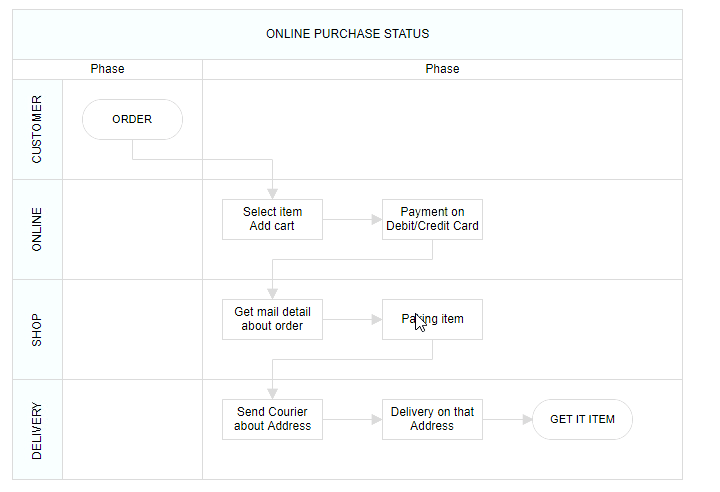Diagram provides the support to edit Lane headers at runtime. The header editing can be achieved by double click event. Double clicking the header label will enable its editing.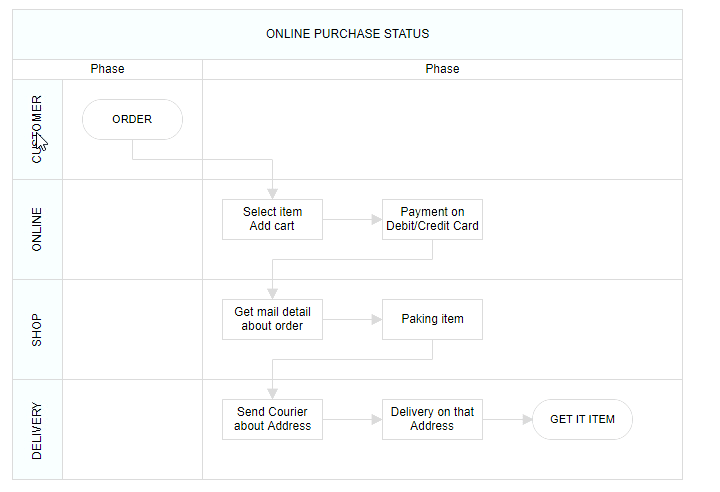## Phase

Phase are the subprocess which will split each lane as horizontally or vertically based on the swimlane orientation. The multiple number of `Phase` can be added to swimlane.

``````<ejs-diagram id="container" width="100%" height="700px">
<e-diagram-nodes>
<e-diagram-node id='node1' offsetX="100" offsetY="100" width="100" height="100" borderWidth="2">
<e-node-style fill="darkcyan", strokeColor="black" strokeWidth="2"></e-node-style>
</e-diagram-node>
</e-diagram-nodes>
</ejs-diagram>``````
``````using System;
using System.Collections.Generic;
using System.Linq;
using System.Web;
using System.Web.Mvc;
using Syncfusion.EJ2.Diagrams;
using System.Drawing;

namespace EJ2MVCSampleBrowser.Controllers.Diagram {
public partial class DiagramController: Controller {
// GET: Nodes
public ActionResult Nodes() {
List < DiagramNode > nodes = new List < DiagramNode > ();
List < DiagramNodeAnnotation > Node1 = new List < DiagramNodeAnnotation > ();
Content = "node1", Style = new DiagramTextStyle() {
Color = "White", StrokeColor = "None"
}
});
List<Lane> Lanes = new List<Lane>();
{
Id = "stackCanvas1",
Height = 100,
{
Annotation = new DiagramNodeAnnotation() { Content = "Consumer" },
Width = 50,Style = new DiagramTextStyle() { FontSize = 11 ,fill ="red"}
},
});
List<Phase> Phases = new List<Phase>();
{
Id= "phase1",
Offset=170,
{
Annotation = new DiagramNodeAnnotation() { Content = "Phase" },
},
});
swimlane.Shape  =  new SwimLane(){
Type = "SwimLane",
PhaseSize = 20,
{
Annotation = new DiagramNodeAnnotation() { Content = "ONLINE PURCHASE STATUS" },
Height = 50,
Orientation = "Horizontal",
Style = new DiagramTextStyle() { FontSize = 11 ,fill ="red"}
},
Lanes = Lanes,
Phases = Phases
};
ViewBag.nodes = nodes;
return View();
}
}
public class Node: DiagramNode {
public string text;
}
}``````

### Dynamically add phase to lane

You can add the phase at runtime by using client side API method called `addPhases`.

``````  let phase = [{
id: 'phase1', offset: 170,
header: { content: { content: 'Phase' } }
}]

### Customizing phase

``````<ejs-diagram id="container" width="100%" height="700px">
<e-diagram-nodes>
<e-diagram-node id='node1' offsetX="100" offsetY="100" width="100" height="100" borderWidth="2">
<e-node-style fill="darkcyan", strokeColor="black" strokeWidth="2"></e-node-style>
</e-diagram-node>
</e-diagram-nodes>
</ejs-diagram>``````
``````using System;
using System.Collections.Generic;
using System.Linq;
using System.Web;
using System.Web.Mvc;
using Syncfusion.EJ2.Diagrams;
using System.Drawing;

namespace EJ2MVCSampleBrowser.Controllers.Diagram {
public partial class DiagramController: Controller {
// GET: Nodes
public ActionResult Nodes() {
List < DiagramNode > nodes = new List < DiagramNode > ();
List < DiagramNodeAnnotation > Node1 = new List < DiagramNodeAnnotation > ();
Content = "node1", Style = new DiagramTextStyle() {
Color = "White", StrokeColor = "None"
}
});
List<Lane> Lanes = new List<Lane>();
{
Id = "stackCanvas1",
Height = 100,
{
Annotation = new DiagramNodeAnnotation() { Content = "Consumer" },
Width = 50,Style = new DiagramTextStyle() { FontSize = 11 ,fill ="red"}
},
});
List<Phase> Phases = new List<Phase>();
{
Id= "phase1",
Offset=170,
{
Annotation = new DiagramNodeAnnotation() { Content = "Phase" },
},
});
swimlane.Shape  =  new SwimLane(){
Type = "SwimLane",
PhaseSize = 20,
{
Annotation = new DiagramNodeAnnotation() { Content = "ONLINE PURCHASE STATUS" },
Height = 50,
Orientation = "Horizontal",
Style = new DiagramTextStyle() { FontSize = 11 ,fill ="red"}
},
Lanes = Lanes,
Phases = Phases
};
ViewBag.nodes = nodes;
return View();
}
}
public class Node: DiagramNode {
public string text;
}
}``````

### Resizing

• The phase can be resized by using its selector.
• You must select the phase header to enable the phase selection.
• Once the phase can be resized, the lane size will be updated automatically.

### Resizing helper

• The special resize selector will be used to resize the phase.
• The resize cursor will be available on the left and bottom direction for horizontal, and the top and bottom direction for vertical swimlane.

Diagram provides the support to edit phase headers at runtime. The header editing is achieved by double click event. Double clicking the header label will enable the editing of that.Diagram provides support to add swimlane and phases to symbol palette.

``````<ejs-diagram id="container" width="100%" height="700px">
<e-diagram-nodes>
<e-diagram-node id='node1' offsetX="100" offsetY="100" width="100" height="100" borderWidth="2">
<e-node-style fill="darkcyan">
</e-node-style>
</e-diagram-node>
</e-diagram-nodes>
</ejs-diagram>``````
``````using System;
using System.Collections.Generic;
using System.Linq;
using System.Web;
using System.Web.Mvc;
using Syncfusion.EJ2.Diagrams;
using System.Drawing;

namespace EJ2MVCSampleBrowser.Controllers.Diagram {
public partial class DiagramController: Controller {
// GET: Nodes
public ActionResult Nodes() {
List < DiagramNode > nodes = new List < DiagramNode > ();
List < DiagramNodeAnnotation > Node1 = new List < DiagramNodeAnnotation > ();
Content = "node1", Style = new DiagramTextStyle() {
Color = "White", StrokeColor = "None"
}
});
Id = "node1",
Width = 100,
Height = 100,
BorderWidth=2,
Style = new NodeStyleNodes() {
Fill = "darkcyan"
},
text = "node1",
OffsetX = 100,
OffsetY = 100,
Annotations = Node1
});
ViewBag.nodes = nodes;

return View();
}
}
public class Node: DiagramNode {
public string text;
}
}``````

### Drag and drop swimlane to palette

• The drag and drop support for swimlane shapes has been provided.
• When you drag and drop the lane shape, if the diagram already contains swimlane with the same orientation, the lane will be added and stacked inside a swimlane based on the order. Otherwise, it will be added a new swimlane.
• The phase will only drop on swimlane shape with same orientation.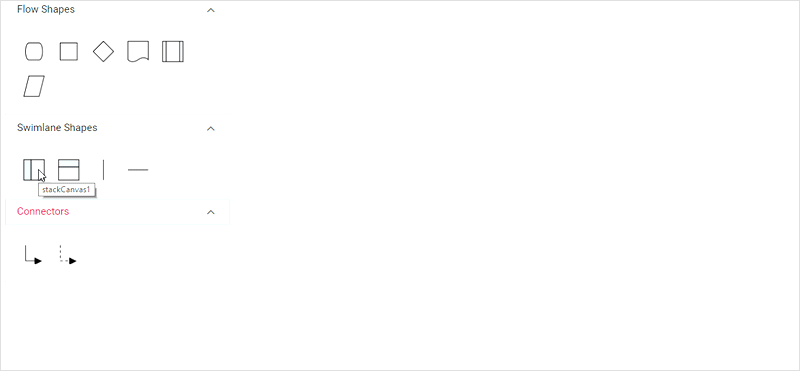## Limitations

• Connectors cannot be canceled when added directly to swimlane. You must initialize the connector through connector collection.
• The phase line style cannot be edited.